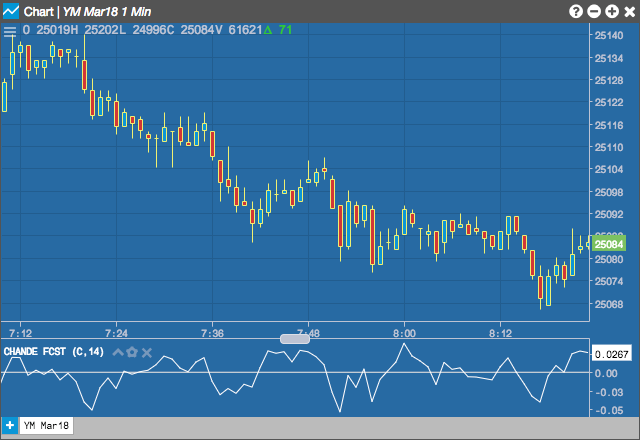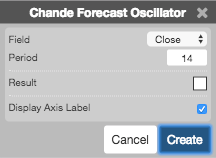Charts

# Chande Forecast Oscillator (CFO)

The Chande Forecast Oscillator plots the percentage difference between the closing price and the n-period linear regression forecasted price. The oscillator is above zero when the forecast price is greater than the closing price and less than zero if it is below.## Configuration Options• Field: Price or combination of prices to use as the base for average calculations. Possible values include:
• Open
• High
• Low
• Close
• HL/2 $$\left ( \frac{High + Low}{2} \right )$$
• HLC/3 $$\left ( \frac{High + Low + Close}{3} \right )$$
• HLCC/4 $$\left ( \frac{High + Low + Close + Close}{4} \right )$$
• OHLC/4 $$\left ( \frac{Open + High + Low + Close}{4} \right )$$
• Period: Number of bars to use in the calculations.
• Color Selectors: Colors to use for graph elements.
• Display Axis Label: Whether to display the most recent value on the Y axis.

## Formula

$CFO_{i} = \frac{(Close_{i} - Linear\;Regression)\times 100}{Close_{i}}$

where linear regresion is calcualted as

$slope\;=\;m\;=\;\frac{\sum_{i=1}^{n}(x_{i} - \bar{x})(y_{i} - \bar{y})}{\sum_{i=1}^{n}(x_{i} - \bar{x})^{2}}$

$intercept\;=\;b\;=\;\bar{y} - m\bar{x}$